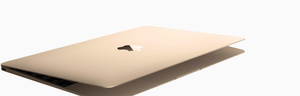# Refurbished Mac

Discover what goes into each refurbished Mac.

A\$969.00

A\$1,099.00

A\$1,209.00

A\$1,349.00

A\$1,359.00

A\$1,359.00

A\$1,359.00

A\$1,489.00

A\$1,609.00

A\$1,679.00

A\$1,679.00

A\$1,679.00

A\$1,699.00

A\$1,699.00

A\$1,739.00

A\$1,739.00

A\$1,739.00

A\$1,949.00

A\$1,949.00

A\$1,999.00

A\$1,999.00

A\$2,209.00

A\$2,359.00

A\$2,379.00

A\$2,459.00

A\$2,509.00

A\$2,549.00

A\$2,549.00

A\$2,679.00

A\$2,759.00

A\$2,799.00

A\$2,799.00

A\$2,799.00

A\$2,909.00

A\$2,909.00

A\$2,919.00

A\$2,919.00

A\$2,969.00

A\$3,059.00

A\$3,059.00

A\$3,059.00

A\$3,069.00

A\$3,179.00

A\$3,279.00

A\$3,309.00

A\$3,309.00

A\$3,309.00

A\$3,309.00

A\$3,319.00

A\$3,419.00

A\$3,419.00

A\$3,449.00

A\$3,559.00

A\$3,559.00

A\$3,569.00

A\$3,569.00

A\$3,569.00

A\$3,569.00

A\$3,669.00

A\$3,729.00

A\$3,799.00

A\$3,819.00

A\$3,909.00

A\$3,959.00

A\$3,999.00

A\$4,079.00

A\$4,079.00

A\$4,099.00

A\$4,099.00

A\$4,209.00

A\$4,269.00

A\$4,329.00

A\$4,349.00

A\$4,349.00

A\$4,509.00

A\$4,539.00

A\$4,539.00

A\$4,639.00

A\$4,639.00

A\$4,639.00

A\$4,639.00

A\$4,759.00

A\$4,759.00

A\$4,819.00

A\$4,839.00

A\$4,889.00

A\$4,889.00

A\$5,049.00

A\$5,079.00

A\$5,189.00

A\$5,189.00

A\$5,299.00

A\$5,319.00

A\$5,439.00

A\$5,439.00

A\$5,459.00

A\$5,589.00

A\$5,709.00

A\$5,869.00

A\$5,999.00

A\$6,119.00

A\$6,119.00

A\$6,169.00

A\$6,249.00

A\$6,249.00

A\$6,859.00

A\$6,859.00

A\$7,089.00

A\$7,639.00

A\$7,879.00

A\$8,139.00

A\$8,389.00